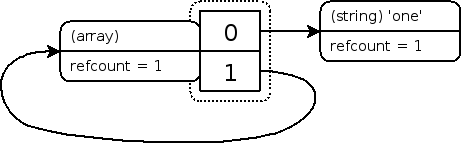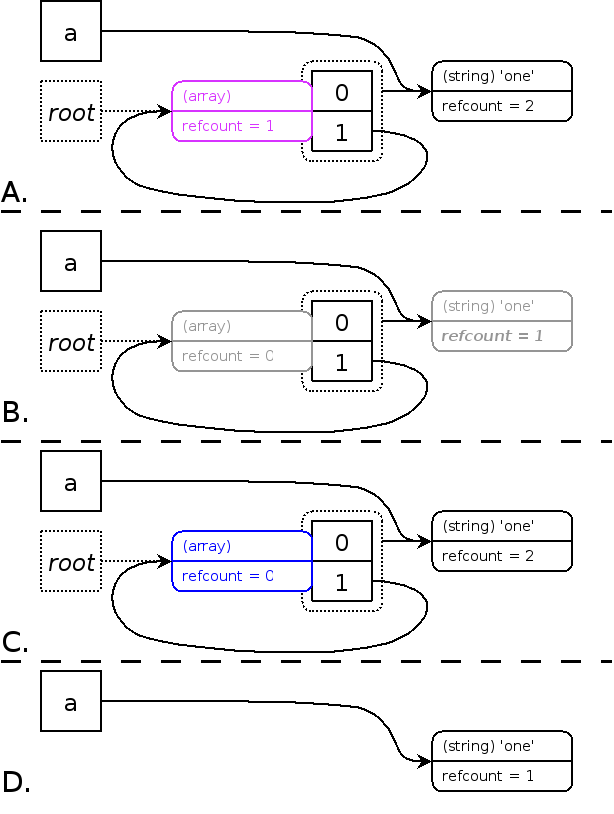PHP 垃圾回收机制

PHP 5.3 之前使用 Reference Counting(引用计数)

zval 是 PHP 存放变量的容器。more
refcount 是计数器，每当有变量引用同一个 zval 变量容器时计数器就会加 1，当取消一个引用时变量就会减 1，当计数器为 0 时，表示这块 zval 内存没有被使用，随即被销毁，完成一次垃圾回收。 more
is_ref 是一个布尔类型，用来标示变量是否被引用。

# refcount 计数器示例
$a = "new string"; xdebug_debug_zval('a'); // 结果: a: (refcount=1, is_ref=0)='new string'$b = $a; xdebug_debug_zval('a'); // 结果: a: (refcount=2, is_ref=0)='new string' unset ($b);
xdebug_debug_zval('a'); // 结果: a: (refcount=1, is_ref=0)='new string'
# is_ref 引用示例
$a = "new string"; xdebug_debug_zval('a'); // 结果: a: (refcount=1, is_ref=0)='new string'$b = & $a; xdebug_debug_zval('a'); // 结果: a: (refcount=2, is_ref=1)='new string' 但是 Reference Counting 存在缺陷，在引用自身 (循环引用) 时会造成内存泄露： $a = [ 'one'];
$a [] =&$a;
xdebug_debug_zval( 'a' );

a: (refcount=2, is_ref=1)=array (
0 => (refcount=1, is_ref=0)='one',
1 => (refcount=2, is_ref=1)=...
)PHP 5.3 之后使用 Collecting Cycles 算法(回收周期)A 步骤把所有可能发生内存泄露的 zval 放入根缓冲区，并确保每个只出现一次。
B 步骤为模拟删除，把所有缓冲区的根 zval 遍历，并将对应的 refcount 减 1，并确保只模拟删除一次。
C 步骤为模拟恢复，把所有缓冲区的根 zval 遍历，找到 refcount 大于 0 的，并将对应的 refcount 加 1。
D 步骤把所有剩余 recount 为 0 的 zval 删除。

两种垃圾回收的区别

• PHP 5.3 之后是当缓冲区满时才进行垃圾回收，5.3 之前是只要某个 zvalrefcount 减为 0 时就回收。
• 由上可知，PHP 5.3 之后的回收算法占用内存，5.3 之前的耗时间。
• 新的垃圾回收机制将内存泄露控制在一个范围 (取决于缓冲区的大小)